International
Tables for
Crystallography
Volume F
Crystallography of biological macromolecules
Edited by M. G. Rossmann and E. Arnold

International Tables for Crystallography (2006). Vol. F, ch. 16.1, pp. 334-335   | 1 | 2 |

Section 16.1.3. Starting the phasing process

G. M. Sheldrick,c H. A. Hauptman,b C. M. Weeks,b* R. Millerb and I. Usóna

aInstitut für Anorganisch Chemie, Universität Göttingen, Tammannstrasse 4, D-37077 Göttingen, Germany,bHauptman–Woodward Medical Research Institute, Inc., 73 High Street, Buffalo, NY 14203-1196, USA, and cLehrstuhl für Strukturchemie, Universität Göttingen, Tammannstrasse 4, D-37077 Göttingen, Germany
Correspondence e-mail:  weeks@orion.hwi.buffalo.edu

16.1.3. Starting the phasing process

| top | pdf |

The phase problem of X-ray crystallography may be defined as the problem of determining the phases ϕ of the normalized structure factors E when only the magnitudesare given. Owing to the atomicity of crystal structures and the redundancy of the known magnitudes, the phase problem is overdetermined and is, therefore, solvable in principle. This overdetermination implies the existence of relationships among the E's and, since the magnitudesare presumed to be known, the existence of identities among the phases that are dependent on the known magnitudes alone. The techniques of probability theory lead to the joint probability distributions of arbitrary collections of E from which the conditional probability distributions of selected sets of phases, given the values of suitably chosen magnitudes, may be inferred.

16.1.3.1. Structure invariants

| top | pdf |

The magnitude-dependent entities that constitute the foundation of direct methods are linear combinations of phases called structure invariants. The term structure invariant' stems from the fact that the values of these quantities are independent of the choice of origin. The most useful of the structure invariants are the three-phase or triplet invariants,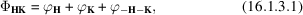the conditional probability distribution (Cochran, 1955), given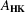, of which is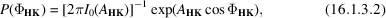where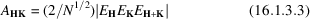and N is the number of atoms, here presumed to be identical, in the asymmetric unit of the corresponding primitive unit cell. This distribution is illustrated in Fig. 16.1.3.1. The expected value of the cosine of a particular triplet,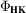, is given by the ratio of modified Bessel functions,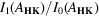.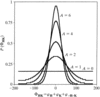Figure 16.1.3.1 | top | pdf |The conditional probability distribution of the three-phase structure invariants.

Estimates of the invariant values are most reliable when the normalized structure-factor magnitudes (,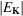and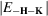) are large and the number of atoms in the unit cell, N, is small. This is the primary reason why direct phasing is more difficult for macromolecules than it is for small molecules. Four-phase or quartet invariants have proven helpful in small-molecule structure determination, particularly when used passively as the basis for a figure of merit (DeTitta et al., 1975). However, the reliability of these invariants, as given by their conditional probability distribution (Hauptman, 1975), is proportional to, and they have not as yet been shown to be useful for macromolecular phasing. The reliability of higher-order invariants decreases even more rapidly as structure size increases.

16.1.3.2. Multisolution' methods and trial structures

| top | pdf |

Successful crystal structure determination requires that sufficient phases be found such that a Fourier map computed using the corresponding structure factors will reveal the atomic positions. It is particularly important that the biggest terms (i.e., largest) be included in the Fourier series. Thus, the first step in the phasing process is to sort the reflections in decreasing order according to theirvalues and to choose the number of largereflections that are to be phased. The second step is to generate the possible invariants involving these intense reflections and then to sort them in decreasing order according to theirvalues. Those invariants with the largestvalues are retained in sufficient number to achieve the desired overdetermination. Ab initio phase determination by direct methods requires not only a set of invariants, the average values of the cosines of which are presumed to be known, but also a set of starting phases. Therefore, the third step in the phasing process is the assignment of initial phase values. If enough pairs of phases,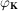and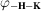, are known, the structure invariants can then be used to generate further phases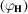which, in turn, can be used to evaluate still more phases. Repeated iterations will permit most reflections with largeto be phased.

Depending on the space group, a small number of phases can be assigned arbitrarily in order to fix the origin position and, in noncentrosymmetric space groups, the enantiomorph. However, except for the simplest structures, these reflections provide an inadequate foundation for further phase development. Consequently, a `multisolution' or multi-trial approach (Germain & Woolfson, 1968) is normally taken in which other reflections are each assigned many different starting values in the hope that one or more of the resultant phase combinations will lead to a solution. Solutions, if they occur, must be identified on the basis of some suitable figure of merit. Although phases can be evaluated sequentially, the order determined by a so-called convergence map (Germain et al., 1970), it has become standard in recent years to use a random-number generator to assign initial values to all available phases from the outset (Baggio et al., 1978; Yao, 1981). A variant of this procedure is to use the random-number generator to assign initial coordinates to the atoms in the trial structures and then to obtain initial phases from a structure-factor calculation.

References

Baggio, R., Woolfson, M. M., Declercq, J.-P. & Germain, G. (1978). On the application of phase relationships to complex structures. XVI. A random approach to structure determination. Acta Cryst. A34, 883–892.Google Scholar
Cochran, W. (1955). Relations between the phases of structure factors. Acta Cryst. 8, 473–478.Google Scholar
DeTitta, G. T., Edmonds, J. W., Langs, D. A. & Hauptman, H. (1975). Use of the negative quartet cosine invariants as a phasing figure of merit: NQEST. Acta Cryst. A31, 472–479.Google Scholar
Germain, G., Main, P. & Woolfson, M. M. (1970). On the application of phase relationships to complex structures. II. Getting a good start. Acta Cryst. B26, 274–285.Google Scholar
Germain, G. & Woolfson, M. M. (1968). On the application of phase relationships to complex structures. Acta Cryst. B24, 91–96.Google Scholar
Hauptman, H. (1975). A new method in the probabilistic theory of the structure invariants. Acta Cryst. A31, 680–687.Google Scholar
Yao, J.-X. (1981). On the application of phase relationships to complex structures. XVIII. RANTAN – random MULTAN. Acta Cryst. A37, 642–644.Google Scholar